Select Page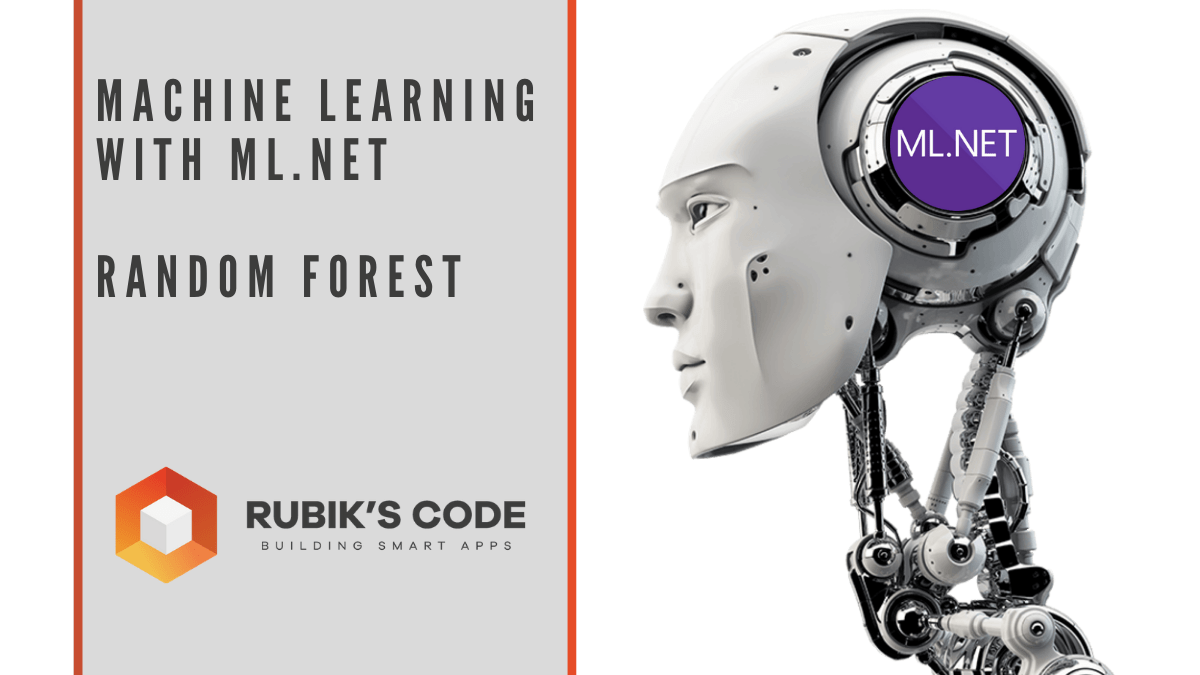## Machine Learning with ML.NET – Random Forest

In a previous couple of articles, we explored some basic machine learning algorithms and how they fit into the .NET world. Thus far we covered some simple regression algorithms, classification algorithms. Apart from that, we learned a bit about unsupervised learning,...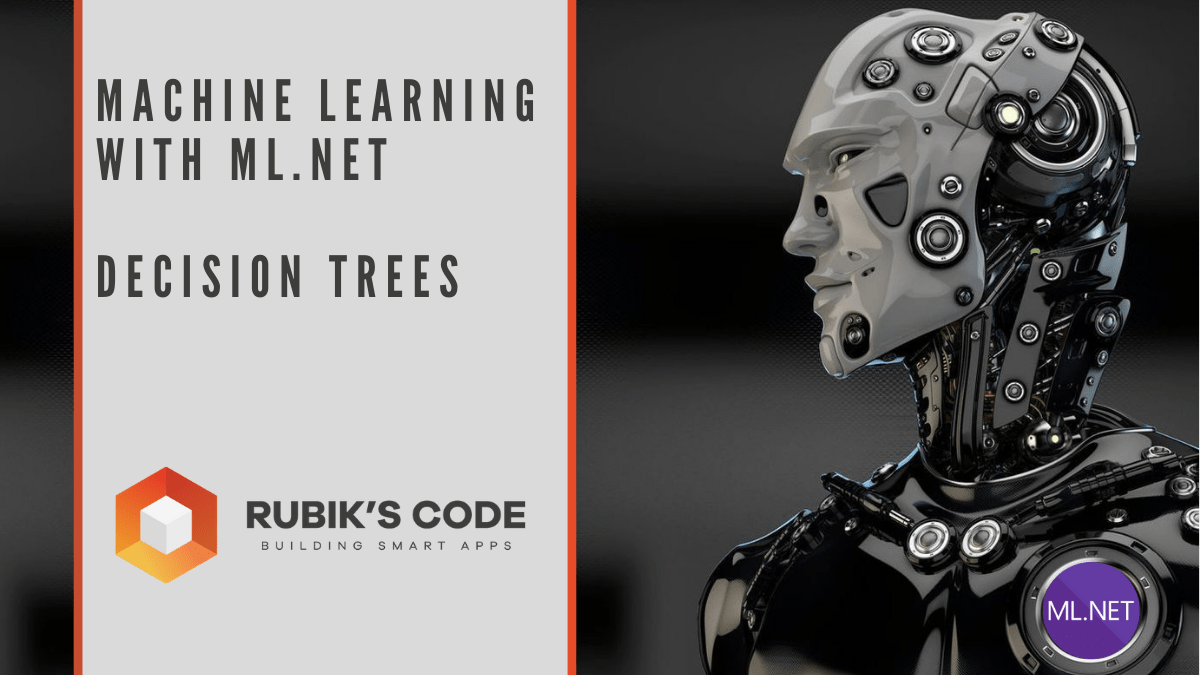## Machine Learning with ML.NET – Guide to Decision Trees

In a previous couple of articles, we explored some basic machine learning algorithms and how they fit into the .NET world. Thus far we covered some simple regression algorithms, classification algorithms. Apart from that, we learned a bit about unsupervised learning,...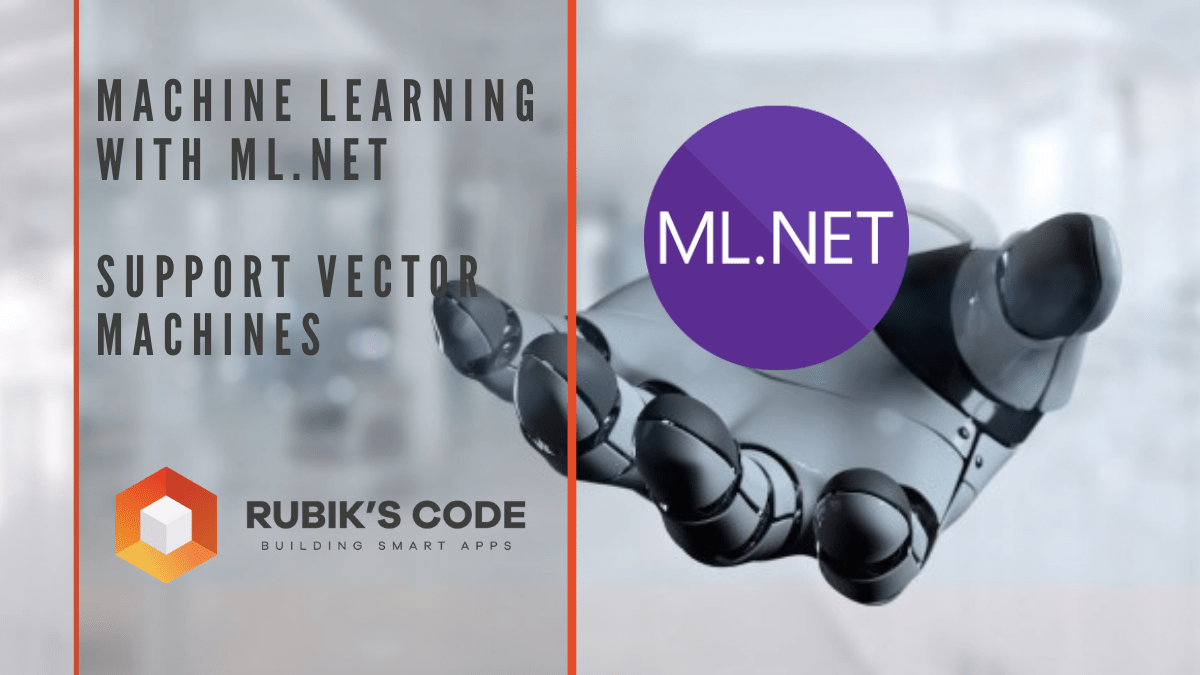## Machine Learning with ML.NET – Support Vector Machines

In a previous couple of articles, we explored some basic machine learning algorithms and how they fit into the .NET world. Thus far we covered some simple regression algorithms, classification algorithms. Apart from that, we learned a bit about unsupervised learning,...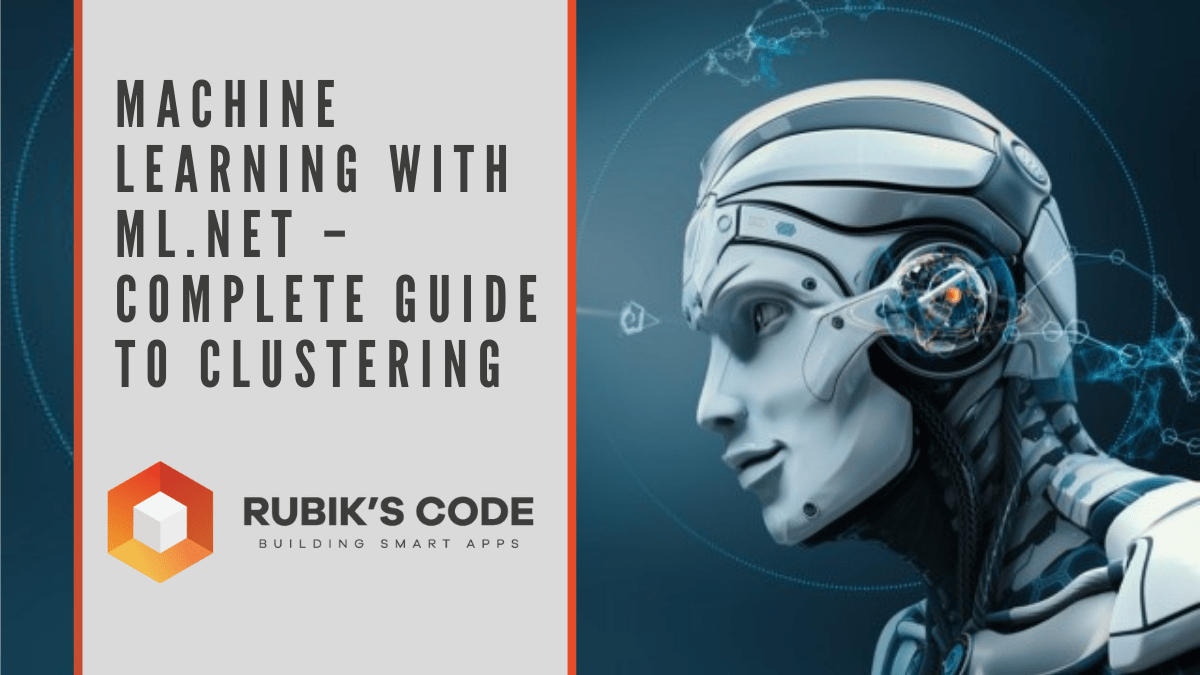## Machine Learning with ML.NET – Complete Guide to Clustering

In a previous couple of articles, we explored some basic machine learning algorithms. Thus far we covered some simple regression algorithms, classification algorithms. We used ML.NET implementation and application of these algorithms. Up to this point, we explored...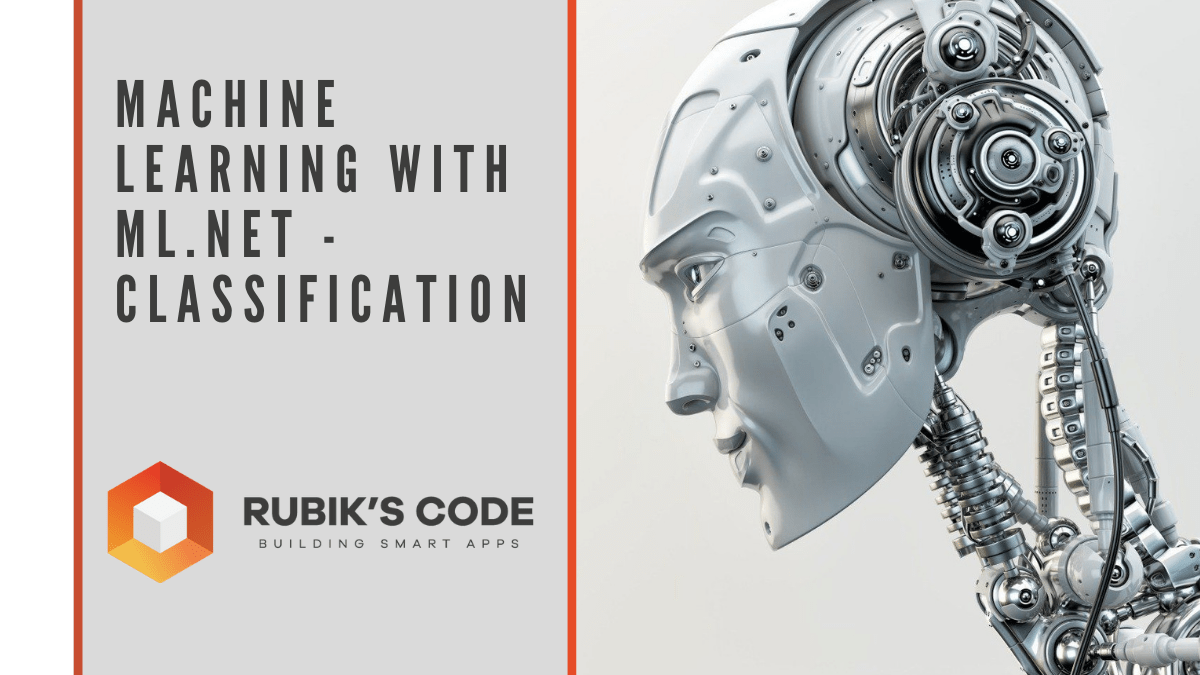## Machine Learning with ML.NET – Ultimate Guide to Classification

In the previous article, we started exploring some of the basic machine learning algorithms and learned how to use ML.NET. There we covered Linear Regression, its variations and we implemented it from scratch with C#. In this article, we focus on the classification...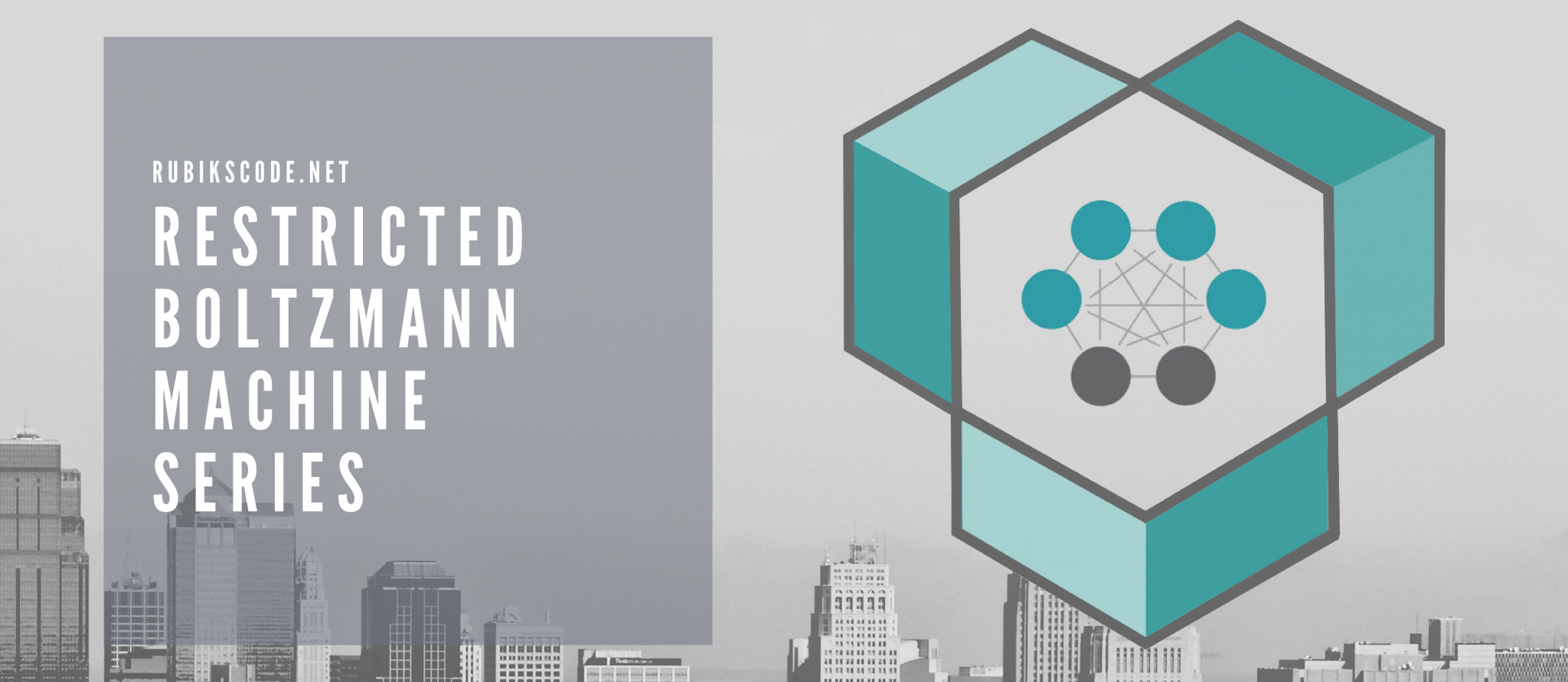## Restricted Boltzmann Machine Series

The path to learning story of Restricted Boltzmann Machine is bumpy. First, we needed to learn what are Energy-Based Models, the group of machine learning models that Restricted Boltzmann Machine is a part of. Then we need to explore functionalities of vanilla...